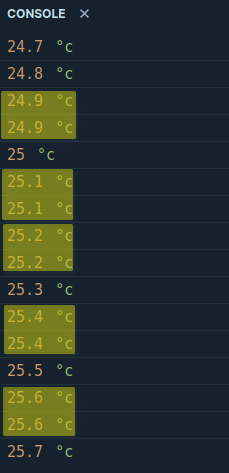# Temperature sensor : is my calcul right?

Temp. calculation :
TEMP (°C) = VAL(DATABYTE1+DATABYTE2)/32 * 0.0625

BYTE1+BYTE2 : \$28 + \$20 => \$2820
division by 32 is similar to bit shift right 5 times (>>)
0.0625 is the resolution of sensor

in the case of \$28 \$20, Temp is 20,06°C

am I right ?Command EA sent to a VMB1TS:

``````ParseTemperature(Data); // Data byte 4 (starting at 0, where byte 0 contains the command)

function ParseTemperature(Value: Byte): Double;
begin
Result := Value;

if Result >= \$80 then
Result := (\$FF - Result + 1) * -1;

Result := Result / 2;
end;``````

I hope never having to use this condition in my house (less than zero degree)
Thanks !

Well, looking at table on VMB1TS protocol… there are 2 BYTES for temperature.
DATABYTE 1
DATABYTE 2 (seems to give resolution on 3 left bits, 5 right bits are always zero).

Temperature isn’t 11 bits ?

golfy,

this is my way to read the temperature from vmbts module

``````           // Get value from VMBTS and put it in waarde(4,5,6)
if (args.Packet.Address == 0x61 && args.Packet.Command == 0xE6) // vmbts on address 61(hex)
{
waarde4 = (((args.Packet * 256) + args.Packet) / 32) * 0.0625;
}
if (args.Packet.Address == 0x62 && args.Packet.Command == 0xE6)// vmbts on address 62(hex)
{
waarde5 = (((args.Packet * 256) + args.Packet) / 32) * 0.0625;
}
if (args.Packet.Address == 0x63 && args.Packet.Command == 0xE6)// vmbts on address 63(hex)
{
waarde6 = (((args.Packet * 256) + args.Packet) / 32) * 0.0625;
}
``````

To sent temperature to vmbts:
private void numericUpDownTemp_leefruimte_ValueChanged(object sender, EventArgs e)
{
packet.Priority = PacketPriority.Low;
packet.Rtr = false;
packet.DataSize = 3;
packet.Command = 0xE4;
packet = 0;
packet = Convert.ToByte(numericUpDownTemp_leefruimte.Value * 2);
packet = 0;
packet = 0;
bus.Send(packet);
}
all this is written in c#.

stis

I was frustrated because I wasn’t able to use the low byte precision of temperature.
Today, I’ve worked a lot to understand, and now, I’m able to get temperature value with 1/10°C.

Here is my JavaScript code :

``````function TempCurrentCalculation(msg) {
// E6 (Transmit Temp) or EA (Sensor status)
switch (msg) {
case 0xE6:
return msg/2 - Math.round(((4-msg)>>5)*0.0625*10)/10
case 0xEA:
return msg/2 - Math.round(((4-msg)>>5)*0.0625*10)/10

default:
console.error("ERROR with TempCalculation",msg)
return undefined
}
}
``````

In fact, the low byte gives values from -0.25 to +0.25 step 0.0625: the real operation is
`((4-msg)>>5)*0.0625`
It works for me, I hope to not commit mistake.

1 Like

For those who want an example, the following code show what happens when HighTemp byte change (50 to 51 give a temperature with 25°C to 25.5°C) while LowTemp byte covers almost all other decimal values.

``````for (let t=0 ; t<8 ; t++) {
r = 25 - Math.round(((4-t))*0.0625*10)/10
console.log(r,"°c")
}
for (let t=0 ; t<8 ; t++) {
r = 25.5 - Math.round(((4-t))*0.0625*10)/10
console.log(r,"°c")
}
``````

Then, the result (some redundancy caused by rounded number):(from my point of view, it’s unnecessary to use more than one decimal number)